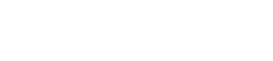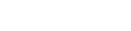Tuesday, 24 August 2021 13:23

## NUMBER PATTERNS, SEQUENCES AND SERIES QUESTIONS AND ANSWERS GRADE 12

Activity 1

1. Given the sequence 6; 13; 20; …
1. Determine a formula for the nth term of the sequence.
2. Calculate the 21st term of this sequence.
3. Determine which term of this sequence is 97. (5)
2. Consider this number pattern: 8; 5; 2; …
1. Calculate the 15th term.
2. Determine which term of this sequence is –289. (4)
3.
1. Given the arithmetic sequence 1 − p; 2p − 3; p + 5; . . . determine the value of p.
2. Determine the values of the first three terms of the sequence. (5)

 Solutions   It is an arithmetic sequence because there is a common difference.a = 6; d = 7 Tn = a + (n – 1)d Tn = 6 + (n – 1)(7)Tn = 7n – 1  T21 = 7(21) – 1 = 147 – 1 = 146  97 = 7n – 1 ∴98 = 7n∴14 = n ∴97 is the 14th term of the sequence. (5)   It is an arithmetic sequence: a = 8; d = 5 – 8 = 2 – 5 = – 3Tn = a + (n – 1)d∴T15 = 8 + (15 – 1)(–3) T15 = 8 + 14(–3)T15 = 8 – 42 = –34  Tn = a + (n – 1)d– 289 = 8 + (n – 1)(–3) ∴– 289 = 8 – 3n +3∴– 300 = –3n∴100 = n 3 ∴– 289 will be the 100th term (4)   Since this is an arithmetic sequence, you can assume that there is a common difference between the terms.d = T2 – T1 = T3 − T2∴(2p – 3) – (1 – p) = ( p + 5) – (2p – 3) 3p – 4 = – p + 8 4p = 12 p = 3T1 = 1 – p = 1 – 3 = –2T2 = 2p – 3 = 2(3) – 3 = 3 T3 = p + 5 = 3 + 5 = 8 So the first three terms of the sequence are –2; 3; 8 (5)

Activity 2

1. Consider the number pattern: 3; 13; 31; 57; 91; …
1. Determine the general term for this pattern.
2. Calculate the 7th term of this pattern.
3. Which term is equal to 241? (9)
2. Find term 6 of this pattern and then find the rule in the form
Tn = an2 + bn + c
–1 ; 3; 9; 17; 27 ... (4)

 Solutions   It helps to make a diagram:∴ it is a quadratic sequence.2a = 8 ∴ a = 4 3a + b = 10 ∴3(4) + b = 10b = –2 a + b + c = 3 ∴ 4 + (–2) + c = 3c = 1 ∴Tn =4n2 – 2n + 1  T7 = 4(7)2 – 2(7) + 1 = 4(49) – 14 + 1= 183  241 = 4n2 – 2n + 10 = 4n2 – 2n + 1 – 241 make the equation = 0 to solve0 = 4n2 – 2n – 2400 = 2n2 – n – 120 divide through by 20 = (2n + 15)(n – 8) factorise∴ 2n + 15 = 0 OR n – 8 = 0∴ n = –7,5 OR n = 8 3 (9)  T1       T2        T3         T4         T5   –1      3         9           17       27 ...          4       6          8          10              2         2           2 ∴T6 = 27 + (10 + 2) = 39 3 use the pattern of the numbers2a = 2 ∴a = 13a + b = 43(1) + b = 4 ∴ b = 1a + b + c = –11 + 1 + c = –1 ∴ c = –3Tn = n2 + n – 3  (4)

n = –7,5 not possible because n is the position of the term so it must be a positive natural number. ✓
∴ 241 is the 8th term of the sequence.

Activity 3

1. Determine the 10th term of the sequence: 3; 6; 12; . . . (2)
2. Determine the number of terms in the sequence: 2; 4; 8; . . .; 1024 (2)
3. If 5; x; 45 are the first three terms of a geometric sequence, determine the value of x. (2)
4. Determine the geometric sequence whose 8th term is 9 and whose 10th term is 25. (3)

 Solutions a = 3; r = 6/3 = 12/6 = 2              Tn = arn−1T10 = 3(2)10−1 = 3(2)9 = 3 × 512 = 1536 (2) a = 2; r = 4/2= 8/4 = 2arn–1 = 10242(2)n–1 = 210 = 2n = 210 ∴ n = 10 (2) x/5 = 45/xx = ± √225 = ± 15 (2) ar7 = 9ar9 = 25ar9 = 25 ar7     9∴r2 = 25/9r = 5/3Activity 4

1. Determine the sum of the series: 19 + 22 + 25 + . . . + 121 (3)
2. The sum of the series 22 + 28 + 34 + . . . is 1870. Determine the number of terms. (2)
3. Given the arithmetic sequence -3; 1; 5; …,393
1. Determine a formula for the nth term of the sequence.
2. Write down the 4th, 5th, 6th and 7th terms of the sequence.
3. Write down the remainders when each of the first seven terms of the sequence is divided by 3.
4. Calculate the sum of the terms in the arithmetic sequence that are divisible by 3. (10)
4. The sum of n terms is given by Sn  = n/2 (1 + n) . Determine T5. (3)
5. 3x + 1; 2x; 3x − 7 are the first three terms of an arithmetic sequence. Calculate the value of x. (3)
6. The first and second terms of an arithmetic sequence are 10 and 6 respectively.
1. Calculate the 11th term of the sequence.
2. The sum of the first n terms of this sequence is –560. Calculate n. (6)

 Solutions a = 19 and d = 3Tn = 3n + 16 = 1213n = 105n = 35Sn  = n/2(a + l)S35  = 35/2 (19 + 121) =  35/2 (140) = 35 × 70 = 2450 (3) a = 22 and d = 6Sn  =  n/2 [2a + (n − 1)d] nn/2 [2 × 22 + (n − 1)6] = 187019n + 3n2  = 18703n2  + 19n − 1870 = 0(3n + 85)(n − 22) = 0 3 ∴ n = 22n cannot be a negative because it is the number of terms (2)   Tn = –3 + (n − 1)4 4n – 7 = Tn  T4  = 5 + 4 = 9; T5  = 9 + 4 = 13; 3 T6  = 13 + 4 = 17 andT7  = 17 + 4 = 21  0; 1; 2; 0; 1; 2; 0 Tn = –3 + 12 (n − 1)393 = 12n – 1512n = 393 + 15 = 408 n = 34S34  = 34/2 × (–3 + 393) = 17 × 390 = 6630 (10) S5  = 5/2 ( 1 + 5 )  = 15 S4  = 4/2 ( 1+ 4 )  = 10 T5 = 15 – 10 = 5 (3) T2 – T1 = T3 – T22x – (3x + 1) = (3x – 7) – 2x 2x – 3x – 1 = 3x – 7 – 2x–2x + 6 = 0 2x = 6x = 3  (3)   Tn = a + (n – 1)dT11 = 10 + (11 – 1)(–4) = –30  Sn =n/2 [2a + (n – 1)d]–560 = n/2 [2(10) + (n – 1)(–4)] –1120 = –4n2 + 24n4n2 – 24n – 1120 = 0n2 – 6n – 280 = 0 (n – 20)(n + 14) = 0 n = 20 or n = –14n = 20 only because number of terms cannot be a negative number (6)

Activity 5

1. Determine 3 + 6 + 12 + 24 + . . . to 10 terms (2)
2. If 2 + 6 + 18 + . . . = 728, determine the value of n. (3)

 Solutions a = 3 and r = 6/3 =  12/6  = 2Sn =  a( r n  − 1)              r - 1S10 = 3( 2 10  − 1) = 3(1024 − 1) = 3069 (2)                2 - 1 a = 2 and r = 6/2  = 18/6 = 3Sn =  2(3n  − 1) = 728               3 - 12(3n  − 1)  = 728      23n − 1 = 7283n = 729 = 36 ∴ n = 6 (3)

Activity 6Look for two different sequences in the pattern and separate them

 Solutions The question asks you to find the sum of the terms from n = 4 ton = 70 if the nth term is 2n – 4.a = T1 = 2(4) – 4 = 4 Find the first term aT2 = 2(5) – 4 = 6T3 = 2(6) – 4 = 8So the sequence is 4; 6; 8; … and this is an arithmetic series. To check d, calculate T2 – T1d = T2 – T1 = 6 – 4 = 2n = (70 – 4) + 1 = 67 There are 67 termsNow we can substitute these values into the formula to find the sum of 67 terms.Sn = n/2 [2a + (n – 1)d]S67 = 67/2 [2(4) + (67 – 1)2]S67 = 33.5 [8 + 132] = 4690(3) This is a geometric series because 5(3)k–1 has the form ark–1, T1 = 5(3)1–1 = 5 ;T2 = 5(3)2–1 = 15; T3 = 5(3)3–1 = 45a = 5; r = 3; n = m and Sm  =  65Sn = a( rn −1)… substitute            r - 165 = 5(3m  − 1) … multiply through by 2              265 = 5(3m  − 1)                2130 = 5.3m – 5 … add like terms135 = 5.3m … divide through by 527 = 3m … write 27 as a power of 333 = 3m … bases are the same, so the powers are equal∴ m = 3 (4)   T1, T3 and T5 form a sequence with a common ratio of ½, so T7 is 1/16 . T2, T4 and T6 form a sequence with a common difference of 3, so T8 is 13. S50 = 25 terms of 1st sequence + 25 terms of 2nd sequenceS50 = ( ½ + ¼ + 1/8 + … to 25 terms) + (4 + 7 + 10 + 13 + … to 25 terms)(5)

Activity 7Solutions T1 = 8(4)1 – 1 = 8 = aTo find r, find the common ratio using T1 and T2, T2 and T3.T2 = 8(4)1 – 2 = 8(4)–1 = 8 × ¼ = 2T3 = 8(4)1 – 3 = 8(4)–2 = 8 × 1/16 = ½T2 ÷ T1 = 2/8 =  ¼  and T3 ÷ T2 =½ = ½ x ½ = ¼                                                     2so r = ¼ and a = 8∴S∞ =   a     =    8    =  8            1 - r      1 - ¼    3/4= 8 x 4/3 = 32/3When dividing by a fraction, you can multiply by the inverse∴S∞ = 32/3 or 102/3 (3) This is a geometric series with r = 2x – 3 To converge –1  <  r  <  1 –1  <  2x – 3  <  1 Add 3 to both sides2 < 2x < 4 Divide by 2 on both sides1 < x < 2 3 x ≠ 3/2 (4)The series will converge for 1 < x < 2 a = 3; r = 2; Sm = 93Sn  =  a(1 −  r n )              1 - r93 = 3 (1 − 2m )            1 - 293 = 3( 1 − 2m)            - 1–93 = 3(1 – 2m) –31 = 1 – 2m2m = 322m = 2 5∴ m = 5 (4) r = 4x – 1 –1 < r < 1-1 < 4x -1 < 1; x ≠ ¼0 < 4x < 20 < x < ½ (3)
Last modified on Wednesday, 25 August 2021 13:36